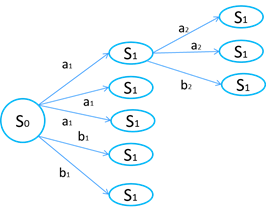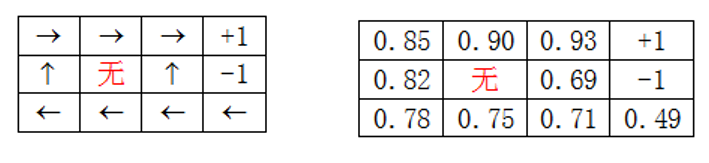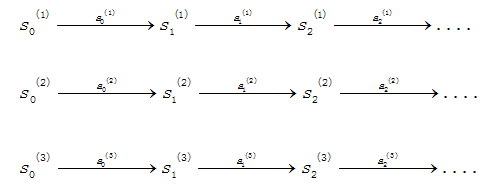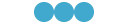# 【机器学习】马尔科夫决策过程A、马尔科夫决策过程

：表示状态集合

：表示一组动作

：表示在某一状态下，采取动作，转移到转态的概率，也就是说在确定的状态下采取相应的动作之后不能完全确定下一状态，而是以一定的概率确定下一状态。

：表示决策过程的一个阻尼系数，用户定义回报在决策过程中随时间打折扣，加快决策国产的收敛

：表示在该状态下的一个回报，有时由动作和状态共同决定回报该时刻的回报1. 将每一个初始状态为s的初始化为0,目标状态累积回报为1

2. 循环直到收敛{

}

1. 同步迭代：即在一次循环过程中，累积回报不更新，而是计算完所有的累积回报之后，再统一更新。

2. 异步迭代，即在一次循环过程中，每计算完一个初始状态下累积回报就立即更新，不需要等到所有的累积回报都计算出来之后再更新。

1. 随机指定一个策略  。

2. 循环直到收敛{

a:令

b:对于每一个状态s，对做更新

}

MDP中的参数估计：1. 随机初始化策略     。

2. 循环直到收敛{

a 在样本上统计该策略下每个状态转移的次数，来估计

b 使用估计到参数来更新对应决策函数下的累积回报

c 根据更新的累积回报重新进行决策，即更新

}

A、马尔可夫决策过程值迭代

/***
马尔科夫决策过程值迭代，关键在于第一次迭代要例外，
因为目标状态是一个终止状态，放到迭代循环里面会出现
临近的状态回报函数无限的，发散。
迭代过程采用的是异步迭代，即每一次内层循环找到更优的
回报就立即更新最大回报，以便与之相邻的状态能立即更新到最优
*/
/****
值迭代
同步更新
12*12*7
*/

while(!flag)
{
flag=1;
for(i=0; i<size; i++)
{
if(action[i]>0||action[i]==0)
maxreward[i]=reward[i]+maxreward[action[i]];
else
maxreward[i]=reward[i];
}//放到这意味着同步更新，count=1008是12*12的7倍，即扫了7遍
for(i=0; i<size; i++)//对每一个状态求最大的V(s)
{
for(j=0; j<size; j++)//策略迭代的话这里其实可以换做扫一遍策略集，这也就是和值迭代不同的地方
{
//cout<<"i="<<i<<" "<<maxreward[i]<<" "<<endl;
if(matrix[i][j]==1&&maxreward[j]>maxreward[i]-reward[i]+0.0001)//更新累积回报
{
action[i]=j;
//if(action[i]>0||action[i]==0)
//maxreward[i]=reward[i]+maxreward[action[i]];//放到这是异步更新，
//else
// maxreward[i]=reward[i];
flag=0;//当累积回报不再更新，即不进入该if，那么就结束迭代
}
count++;
}
}
}

B、马尔可夫决策过程策略迭代

while(!flag)
{
flag=1;
for(i=0; i<size; i++)//对每一个状态求最大的V(s)
{
for(j=0; j<ACTION; j++)//策略迭代的话这里其实可以换做扫一遍策略集，这也就是和值迭代不同的地方
{
//cout<<"i="<<i<<" "<<maxreward[i]<<" "<<endl;
if(matrix[i][ac[j]+i]==1&&maxreward[ac[j]+i]>maxreward[i]-reward[i]+0.0001)
{
action[i]=j;
//if(reward[i]!=1&&reward[i]!=-1)
maxreward[i]=reward[i]+maxreward[ac[j]+i];
//else
// maxreward[i]=reward[i];
flag=0;
}
count++;
}
}
}

C、马尔可夫决策过程动态规划版

/**
4 非递归动态规划
从最终状态出发，采用广度遍历不断的更新其上一状态的累积回报
*/
/*

while(q!=NULL)//这里图的广度遍历没有用到队列，但也用到了队列的思想
//对于当前步能到达的节点用链表连接起来，然后逐渐进行下一步的能到达的节点进行入链（入队列），同样是一种先进先出思想
{
for(i=0; i<size; i++) //由于不是策略迭代，只能遍历所有的状态，找出能到的，且更优的
{
if(matrix[i][q->data]==1&&maxreward[i]<0)//double类型比较大小的偏差，加上一个小数作为精度
{
maxreward[i]=reward[i]+maxreward[q->data];
p=(subset *)malloc(sizeof(subset)*1);
p->data=i;
p->next=NULL;
q=maxsubset;
while((q->next)!=NULL)
q=q->next;
q->next=p;
}
count++;
}
maxsubset->next=maxsubset->next->next;//删除当前节点，即当前步下能到达的节点都已经走完了，可出队列了
q=maxsubset->next;//
}

The EndAI小白入门

ID:StudyForAI©️2019 CSDN 皮肤主题: 大白 设计师: CSDN官方博客